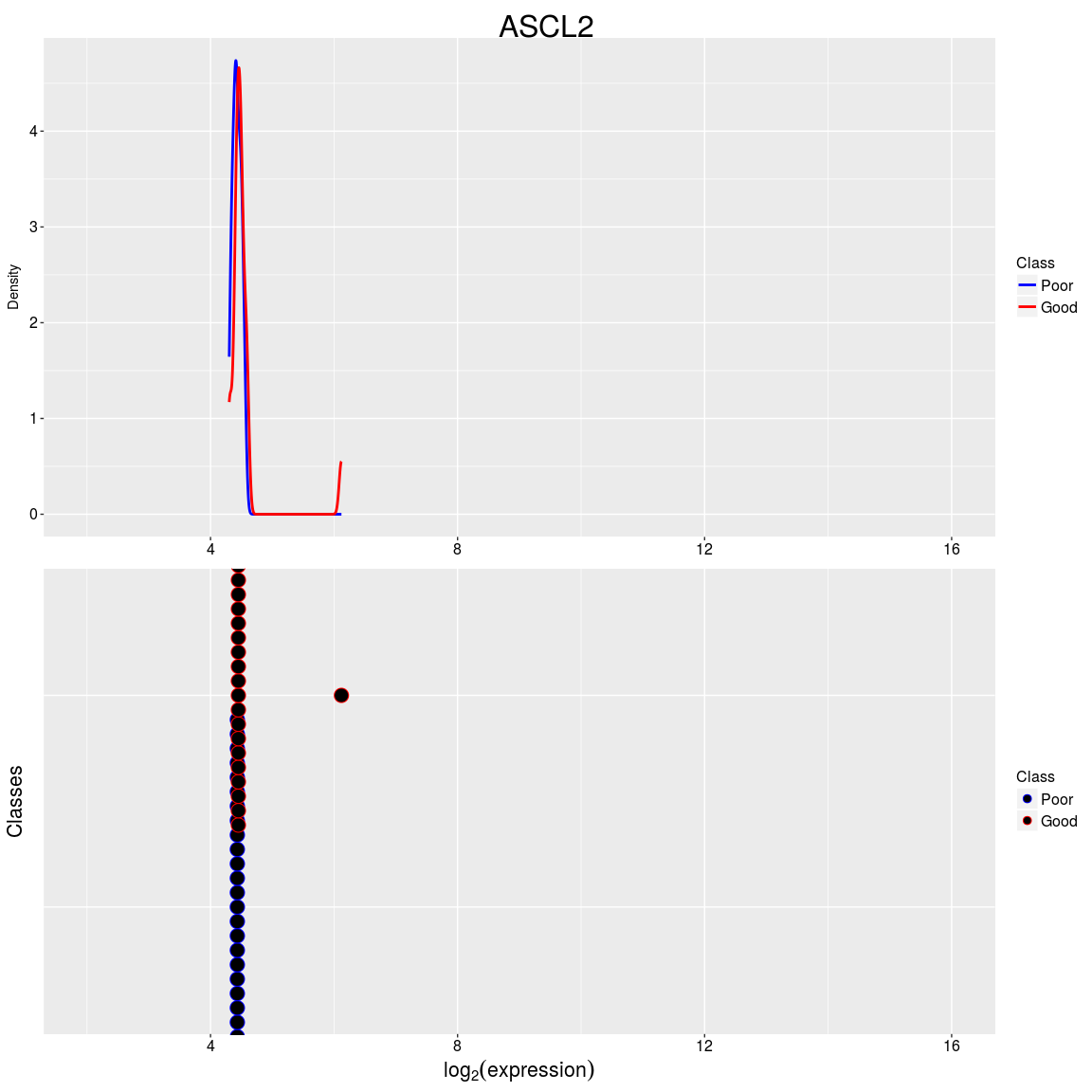# Contents

## 0.1 Overview

ClassifyR provides two contributions. Firstly, there is a structured pipeline for two-class classification. Classification is viewed in terms of four stages, data transformation, feature selection, classifier training, and prediction. The stages can be run in any order that is sensible. Each step can be provided with functions that follow some rules about parameters. Additionally, the driver function implements ordinary k-fold cross-validation, resampling with replacement followed by k-fold or x% test set cross-validation, and leave k out cross-validation. This function can use parallel processing capabilities in R to speed up cross-validations when many CPUs are available. Some convenience function interfaces are provided for microarray and RNA-seq data, while other functions work directly with the framework without the need for an interface.

Secondly, it implements a number of methods for classification using different feature types. Most classifiers work with features where the means are different. In addition to differential expression, ClassifyR also considers differential deviation and differential distribution.

The function that drives the classification is runTests. For cross-validation, it repeatedly calls runTest, which runs a classification for a single split of the data.

In the following sections, the functions provided in ClassifyR will be demonstrated. However, a user can provide any function to the classification framework, as long as it meets some minimal rules. See the last section “Rules for New Functions” for a description of these.

## 0.2 Comparison to Existing Classification Frameworks.

There are a few other frameworks for classification in R. The table below provides a comparison of which features they offer.

Package Run User-defined Classifiers Parallel Execution on any OS Parameter Tuning Calculate over 20 Performance Metrics Ranking and Selection Plots Class Distribution Plot Error Heatmap
ClassifyR Yes Yes Yes Yes Yes Yes Yes
caret Yes Yes Yes No No No No
MLInterfaces Yes No No No No No No
MCRestimate Yes No Yes No No No No
CMA No No Yes No No No No

## 0.3 Case Study : Survival for Ovarian Cancer Patients.

A survival study was performed on microarrays for ovarian cancers and is available from curatedOvarianData on Bioconductor. Load the dataset into the current R session. Only 1000 genes are used for illustration.

``````library(ClassifyR)
library(ggplot2)
library(curatedOvarianData)
data(GSE26712_eset)
GSE26712_eset <- GSE26712_eset[1:1000, ]``````

Define patients who died less than 1 years as poor outcomes, and those that survived more than 5 years as good outcomes.

``````curatedClinical <- pData(GSE26712_eset)
ovarPoor <- curatedClinical[, "vital_status"] == "deceased" & curatedClinical[, "days_to_death"] < 365 * 1
ovarGood <- curatedClinical[, "vital_status"] == "living" & curatedClinical[, "days_to_death"] > 365 * 5
sum(ovarPoor, na.rm = TRUE)
sum(ovarGood, na.rm = TRUE)``````
``````##  27
##  20``````

There are 27 poor prognosis patients and 20 good prognosis patients. The expression data is subset to only keep patients in the Poor or Good group.

``````ovarExpression <- exprs(GSE26712_eset)[, c(which(ovarPoor), which(ovarGood))]
ovarGroups <- factor(rep(c("Poor", "Good"), c(length(which(ovarPoor)), length(which(ovarGood)))),
levels = c("Poor", "Good"))``````

Boxplots are drawn to get an idea of the distrbution of the data.

``````plotData <- data.frame(expression = as.numeric(ovarExpression),
sample = factor(rep(1:ncol(ovarExpression), each = nrow(ovarExpression))))

ggplot(plotData, aes(x = sample, y = expression)) + geom_boxplot() +
scale_y_continuous(limits = c(0, 15)) + xlab("Sample") + ylab("Expression Value") +
ggtitle("Expression for All Arrays") ``````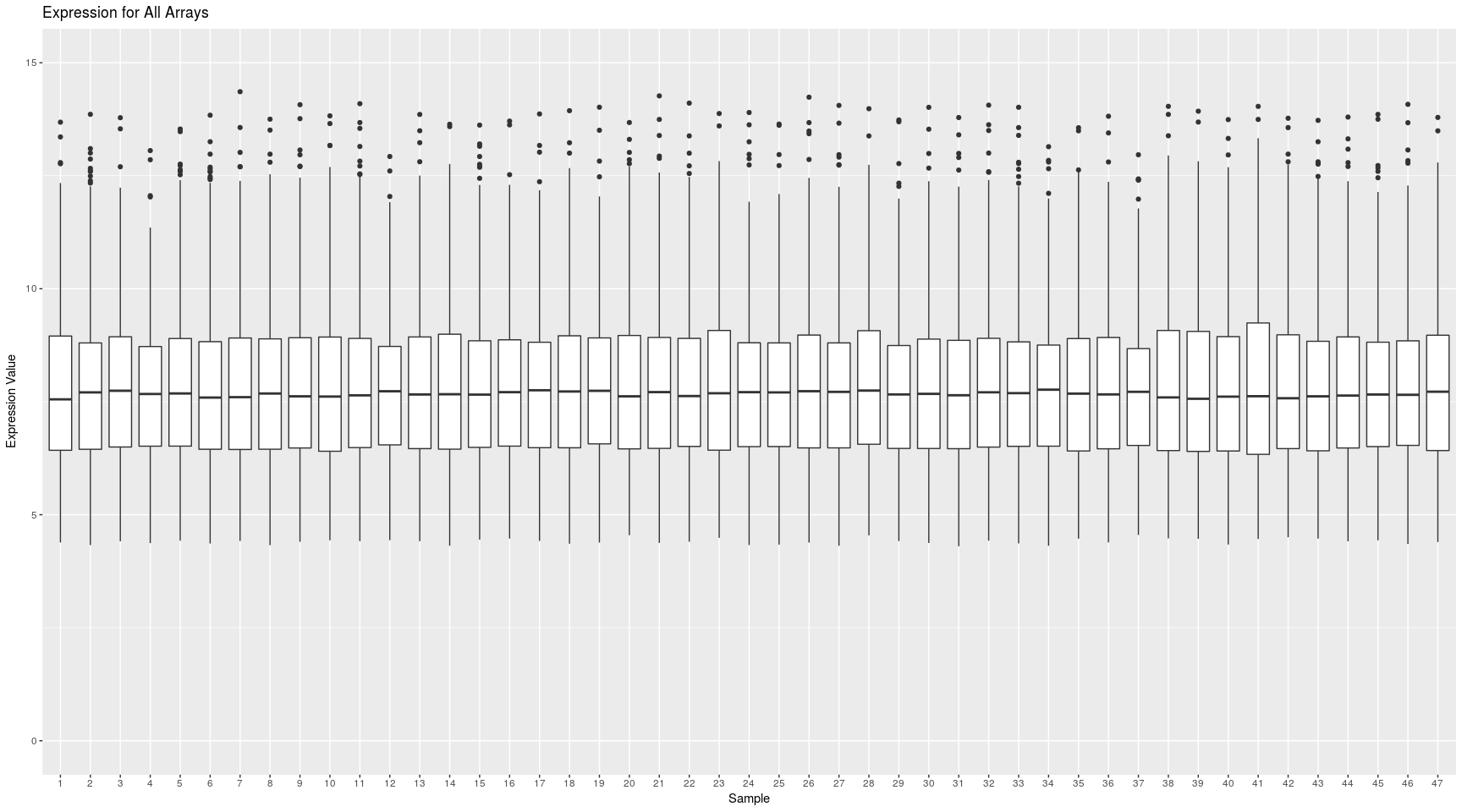All functions provided in ClassifyR work with either a matrix and class vector or an ExpressionSet object. Here, an ExpressionSet object is used.

``````groupsTable <- data.frame(class = ovarGroups)
rownames(groupsTable) <- colnames(ovarExpression)
ovarSet <- ExpressionSet(ovarExpression, AnnotatedDataFrame(groupsTable))
featureNames(ovarSet) <- rownames(ovarExpression)
dim(ovarSet)``````
``````## Features  Samples
##     1000       47``````

### 0.3.1 Differential Expression

Differential expression classifiers look for consistent changes in means between groups. This is the most common form of classification.

Interfaces to existing feature selection and classification algorithms for this type of change included are:

• limmaSelection
• edgeRselection
• nearestShrunkenCentroidSelectionInterface
• nearestShrunkenCentroidTrainInterface
• nearestShrunkenCentroidPredictInterface
• classifyInterface (for Poisson LDA).

limmaSelection is suited to microarray data and edgeRselection is suited to RNA-seq data where the expression values are raw counts.

Here, a feature selection based on a ranked list from limma followed by a DLDA classifier will be used to do 10 resamples and four folds of cross-validation. The dlda function is directly used from the sparsediscrim package in the ClassifyR framework, without any interface being necessary.

``````library(sparsediscrim)
DEresults <- runTests(ovarSet, "Ovarian Cancer", "Differential Expression", validation = "bootstrap", resamples = 5, folds = 3,
params = list(SelectParams(limmaSelection, resubstituteParams = ResubstituteParams(nFeatures = c(25, 50, 75, 100), performanceType = "balanced", better = "lower")),
TrainParams(dlda, TRUE, doesTests = FALSE),
PredictParams(predict, TRUE, getClasses = function(result) result[["class"]])),
parallelParams = bpparam(), verbose = 1)
DEresults``````
``````## An object of class 'ClassifyResult'.
## Dataset Name: Ovarian Cancer.
## Classification Name: Differential Expression.
## Feature Selection Name: Moderated t-test.
## Features: List of length 5 of lists of length 3 of row indices.
## Validation: 5 Resamples, 3 Folds.
## Predictions: List of data frames of length 5.
## Performance Measures: None calculated yet.``````

For computers with more than 1 CPU, the number of cores to use can be given to runTests by using the argument parallelParams.

This example introduces the classes SelectionParams, TrainParams, and PredictParams. They store details about the functions and the parameters they use for selection, training, and prediction. The first argument to their constructors is always a function, followed by other arguments. Any named arguments can be provided, if the function specified to the constructor knows how to use an argument of that name. The order in which they are specified in the list determines the order the stages are run in.

The limmaSelection function specified to selectionParams ranks probes based on p-value and uses the classifier specified for trainParams and calculates the resubstitution error rate for the top nFeatures, picking the value with the lowest error rate.

TrainParams has four mandatory arguments. The first is the function that trains a classifier. The second is a logical value that specifies whether expression should be transposed, before being passed to the classifier function. Many classification functions in existing R packages in the CRAN repository need the features to be the columns and samples to be the rows. In ClassifyR, the expression data that is passed to runTests or runTest must have features as rows and samples as columns. This is more common in bioinformatics. In this example, the function dlda expects columns to be features, so transposeExpression is TRUE. Another common difference between classifiers on CRAN is that some of them do training and testing separately, whereas in other packages, one function does training and testing. In the case of dlda, it only does training, so doesTests, the third argument to the constructor, is set to FALSE.

PredictParams has three mandatory arguments. The first is a function which takes a built classifier and does predictions on unseen data. The second is a function which extracts a vector of predicted class labels, from the object returned from the function. In this case, the predict method returns an object which stores predictions in a list element called class. Additionally, transposeExpression is mandatory. Like for TrainParams, it specifies whether the numeric measurements need to be transposed.

The top five probes selected in the feature selection step can be checked visually. DEresults is a ClassifyResult object returned by runTests. features is a function that allows access to the row indices that were chosen for each fold.

``DEplots <- plotFeatureClasses(ovarSet, features(DEresults)[][][1:5])``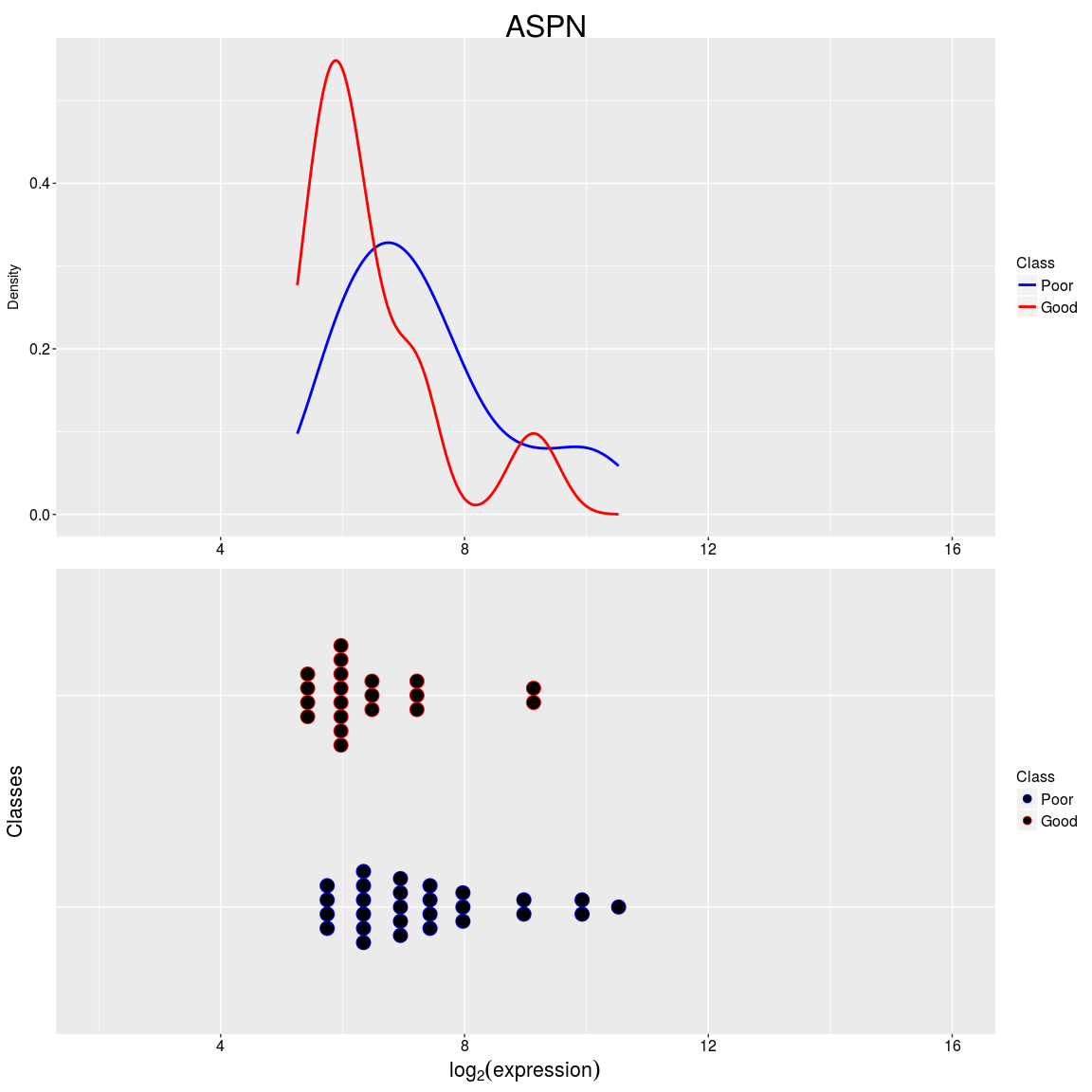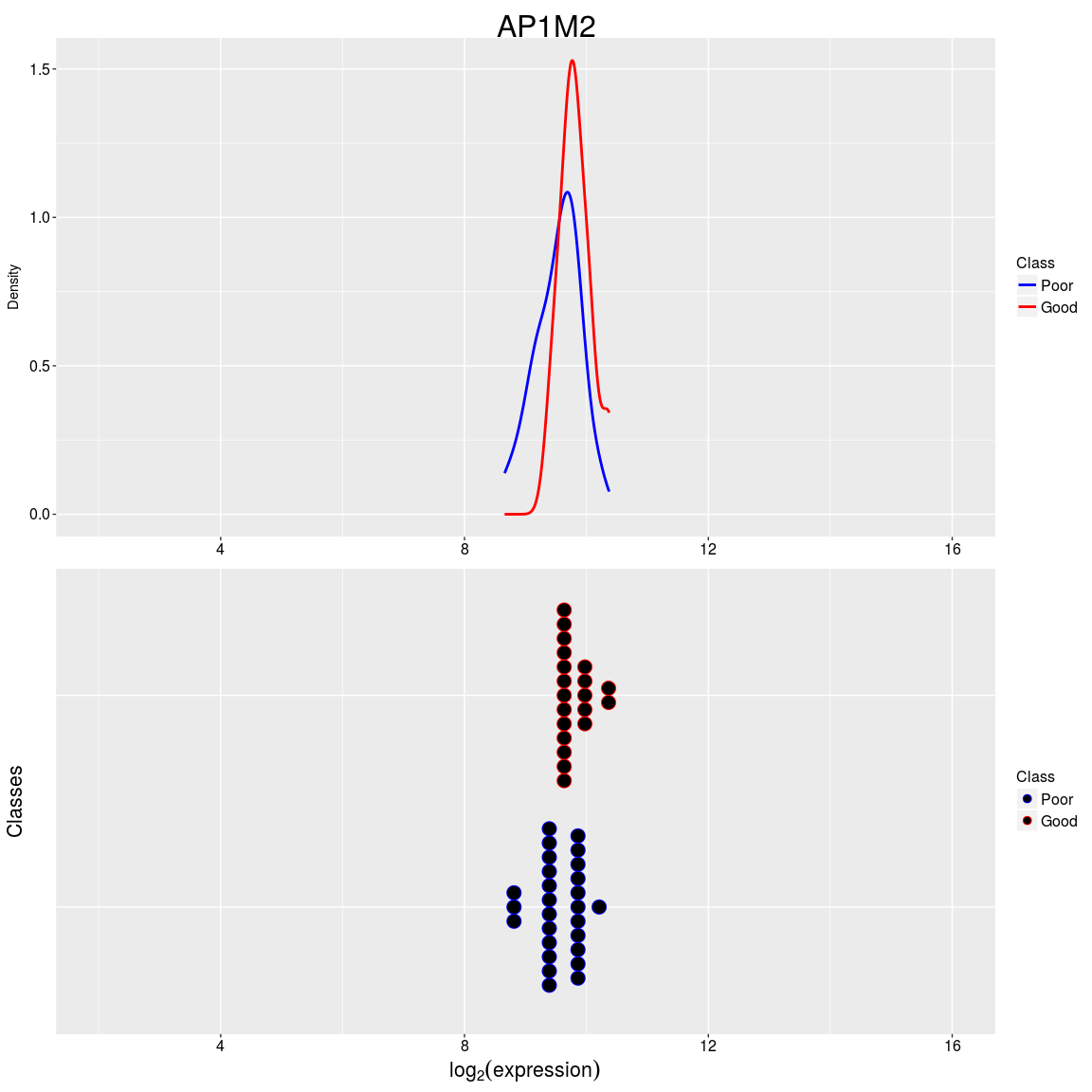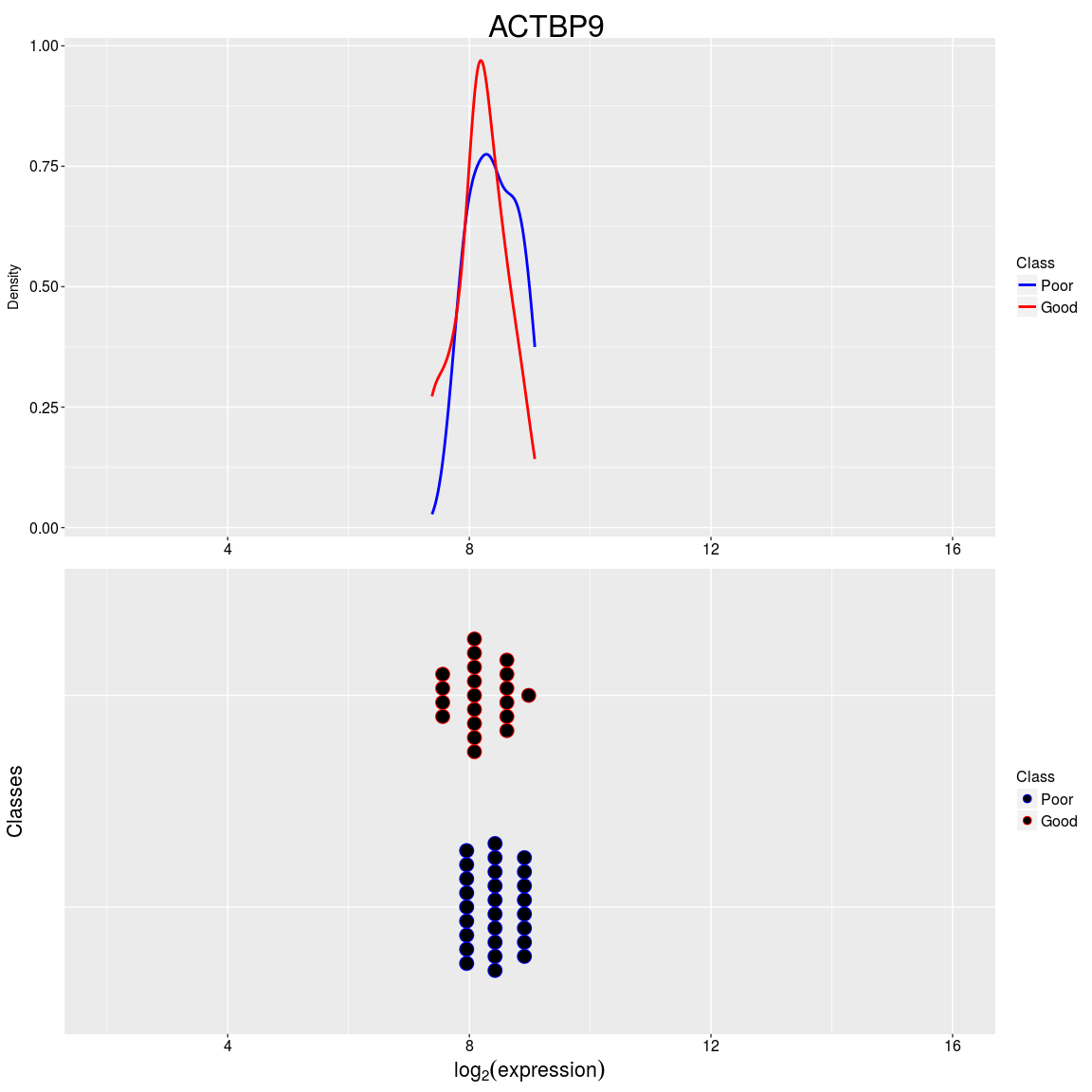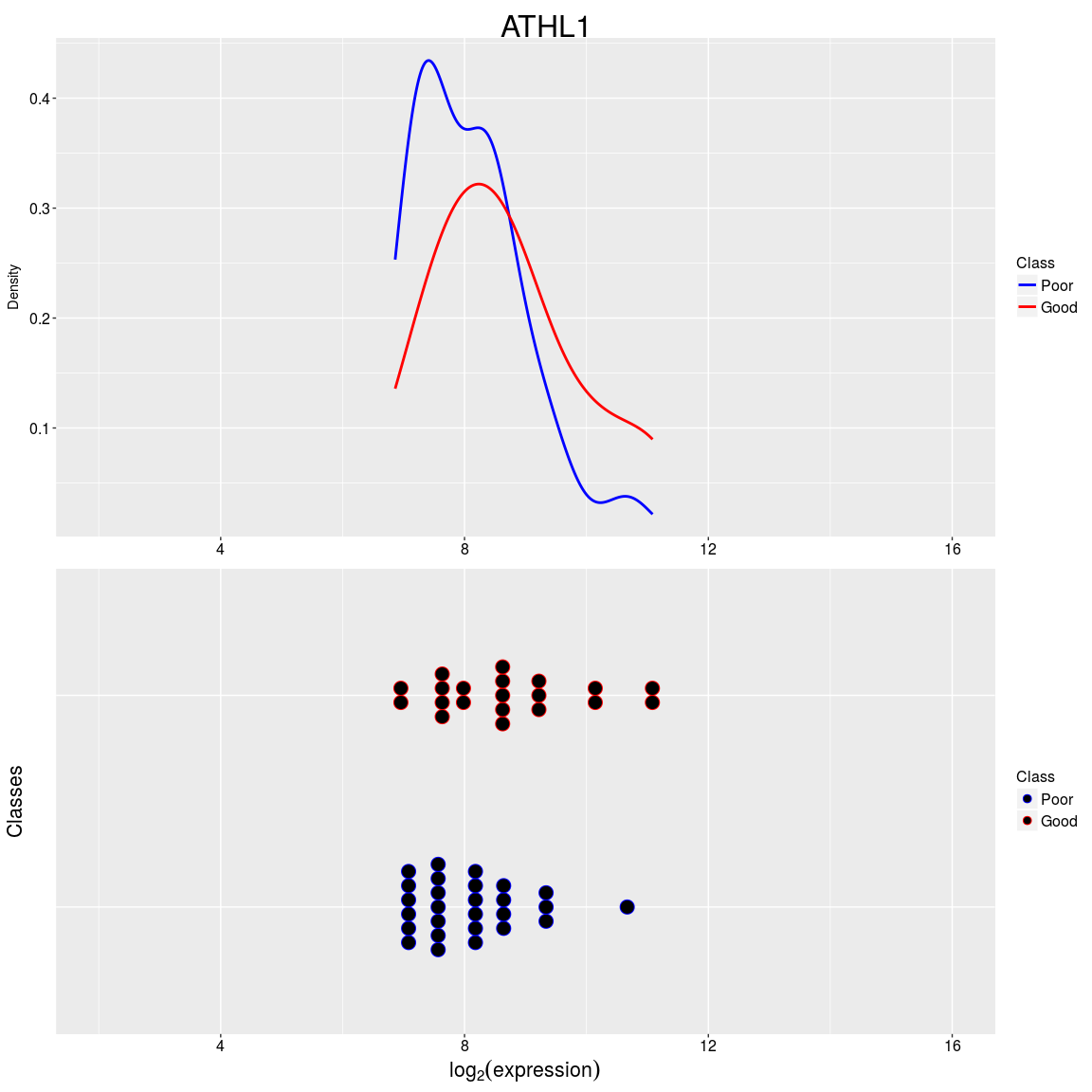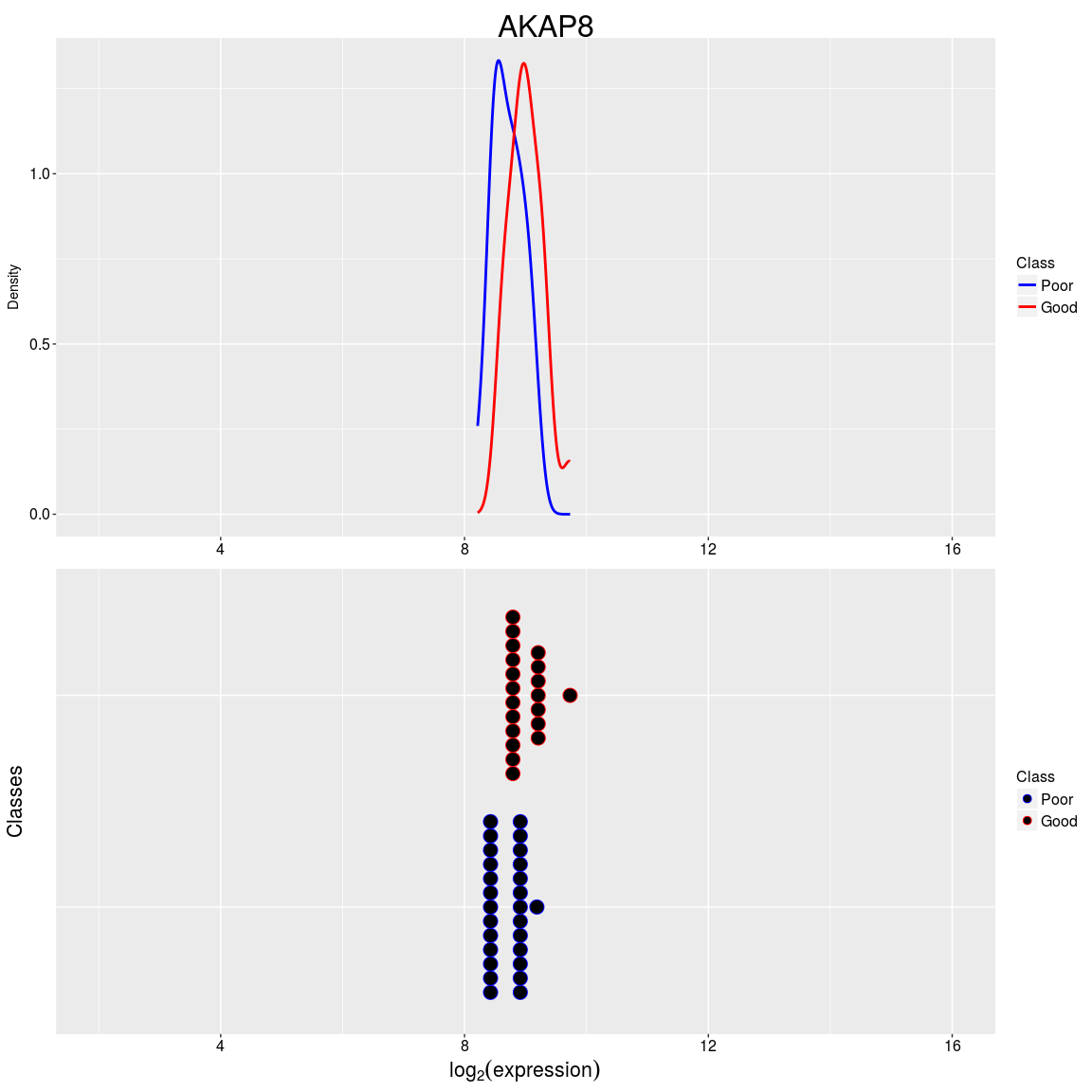This plots the distribution of microarray intensities for the two survival classes for features that were chosen in the first fold of the first resampling. As seen, the means of the probes are not much different.

Classification error rates, as well as many other prediction performance measures, can be calculated with calcPerformance. Next, the balanced error rate is calculated for all ten resamplings. The balanced error rate is defined as the average of the classification errors of each class.

``````DEresults <- calcPerformance(DEresults, "balanced")
DEresults``````
``````## An object of class 'ClassifyResult'.
## Dataset Name: Ovarian Cancer.
## Classification Name: Differential Expression.
## Feature Selection Name: Moderated t-test.
## Features: List of length 5 of lists of length 3 of row indices.
## Validation: 5 Resamples, 3 Folds.
## Predictions: List of data frames of length 5.
## Performance Measures: Balanced Error Rate.``````
``performance(DEresults)``
``````## \$`Balanced Error Rate`
##  0.3357143 0.2250000 0.2565789 0.5018182 0.4111722``````

The error rates are reasonable. Any performance measure can be calculated that the ROCR function performance calculates. For example, the Matthews correlation coefficient can also be calculated.

``````DEresults <- calcPerformance(DEresults, "mat")
DEresults``````
``````## An object of class 'ClassifyResult'.
## Dataset Name: Ovarian Cancer.
## Classification Name: Differential Expression.
## Feature Selection Name: Moderated t-test.
## Features: List of length 5 of lists of length 3 of row indices.
## Validation: 5 Resamples, 3 Folds.
## Predictions: List of data frames of length 5.
## Performance Measures: Balanced Error Rate, Matthews correlation coefficient.``````
``performance(DEresults)``
``````## \$`Balanced Error Rate`
##  0.3357143 0.2250000 0.2565789 0.5018182 0.4111722
##
## \$`Matthews correlation coefficient`
##   0.328571429  0.518544973  0.480560008 -0.003776275  0.178639927``````

### 0.3.2 Differential Variability

Some diseases are typified not by a change in expression means of features between groups, but a change in the expression variability. This can be observed by when the variance of a gene’s expression changes drastically between conditions, such as healthy cells and cancerous cells.

Interfaces to existing feature selection and classification algorithms for this type of change included are:

• leveneSelection
• fisherDiscriminant

Fisher’s LDA is suitable for the absolute value of expression values subtracted from a location, because it does not assume normality, unlike ordinary LDA. Fisher’s LDA is applied to the ovarian cancer data. Only two resamples are done.

``````DVresults <- runTests(ovarSet, "Ovarian Cancer", "Differential Variability",
validation = "bootstrap", resamples = 2, folds = 4,
params = list(SelectParams(leveneSelection, resubstituteParams = ResubstituteParams(nFeatures = c(25, 50, 75, 100), performanceType = "balanced", better = "lower")),
TransformParams(subtractFromLocation, location = "median"),
TrainParams(fisherDiscriminant, FALSE, doesTests = TRUE),
PredictParams(predictor = function(){}, FALSE, getClasses = function(result) result, returnType = "both")),
verbose = 1)
DVresults``````
``````## An object of class 'ClassifyResult'.
## Dataset Name: Ovarian Cancer.
## Classification Name: Differential Variability.
## Feature Selection Name: Levene Test.
## Features: List of length 2 of lists of length 4 of row indices.
## Validation: 2 Resamples, 4 Folds.
## Predictions: List of data frames of length 2.
## Performance Measures: None calculated yet.``````

For params, the SelectionParams object is specified first. In this analysis, feature selection is done first. A TransformParams object is next in the list, so data transformation will be applied after feature selection has been done. transformParams specifies subtractFromLocation as the transformation funcion. This is because it is anticipated that subtracting all features from the median of the training set will be a good feature to detect differential deviation. trainParams specifies fisherDiscriminant as the classifier function. Note that this function does both training and prediction, so the third parameter is TRUE. predictParams specifies an empty function as the prediction function, because fisherDiscriminant does both steps. fisherDiscriminant directly returns a vector of predictions, so the second function simply returns the argument result.

The top five probes selected for the first resampling and first fold are visualised.

``DVplots <- plotFeatureClasses(ovarSet, features(DVresults)[][][1:5])``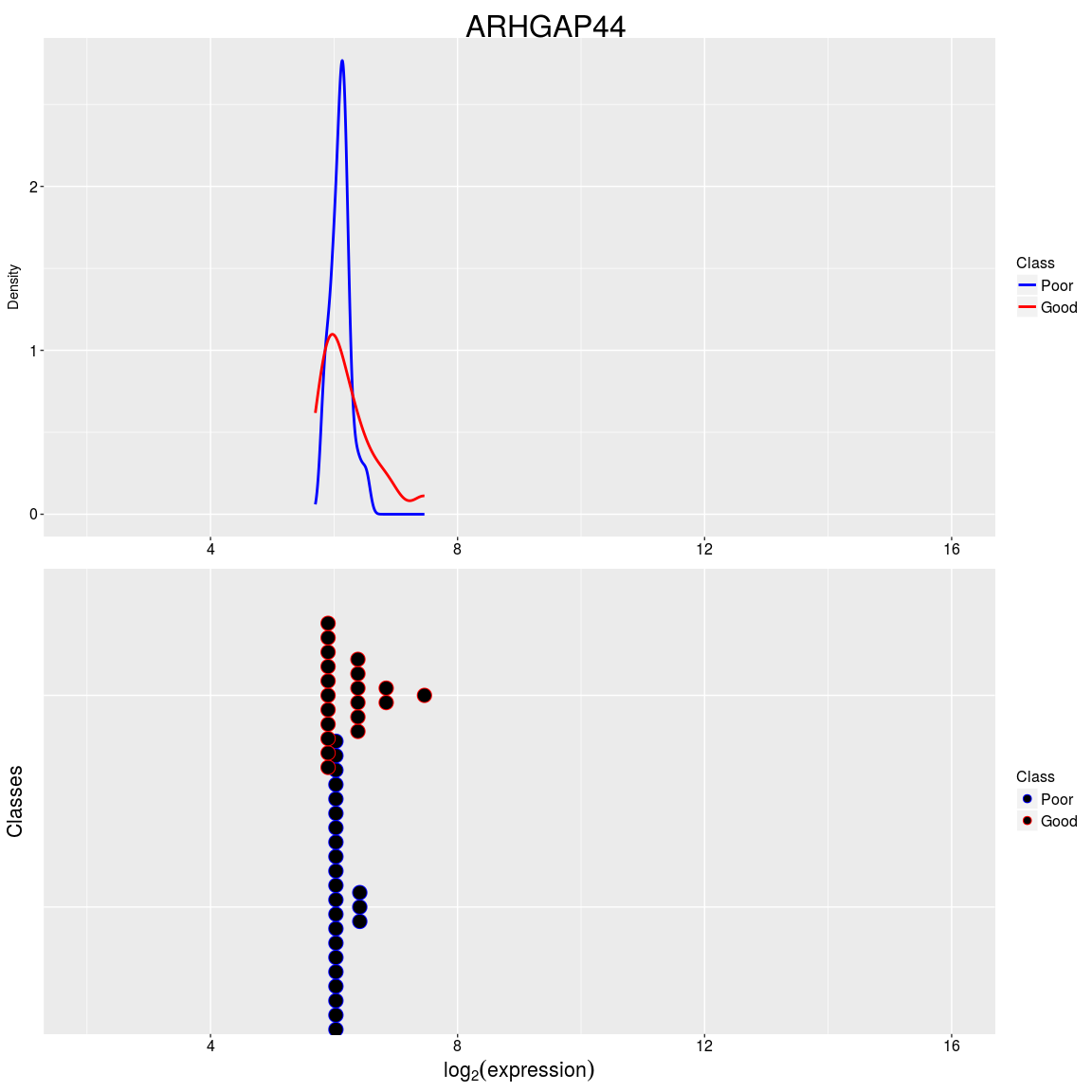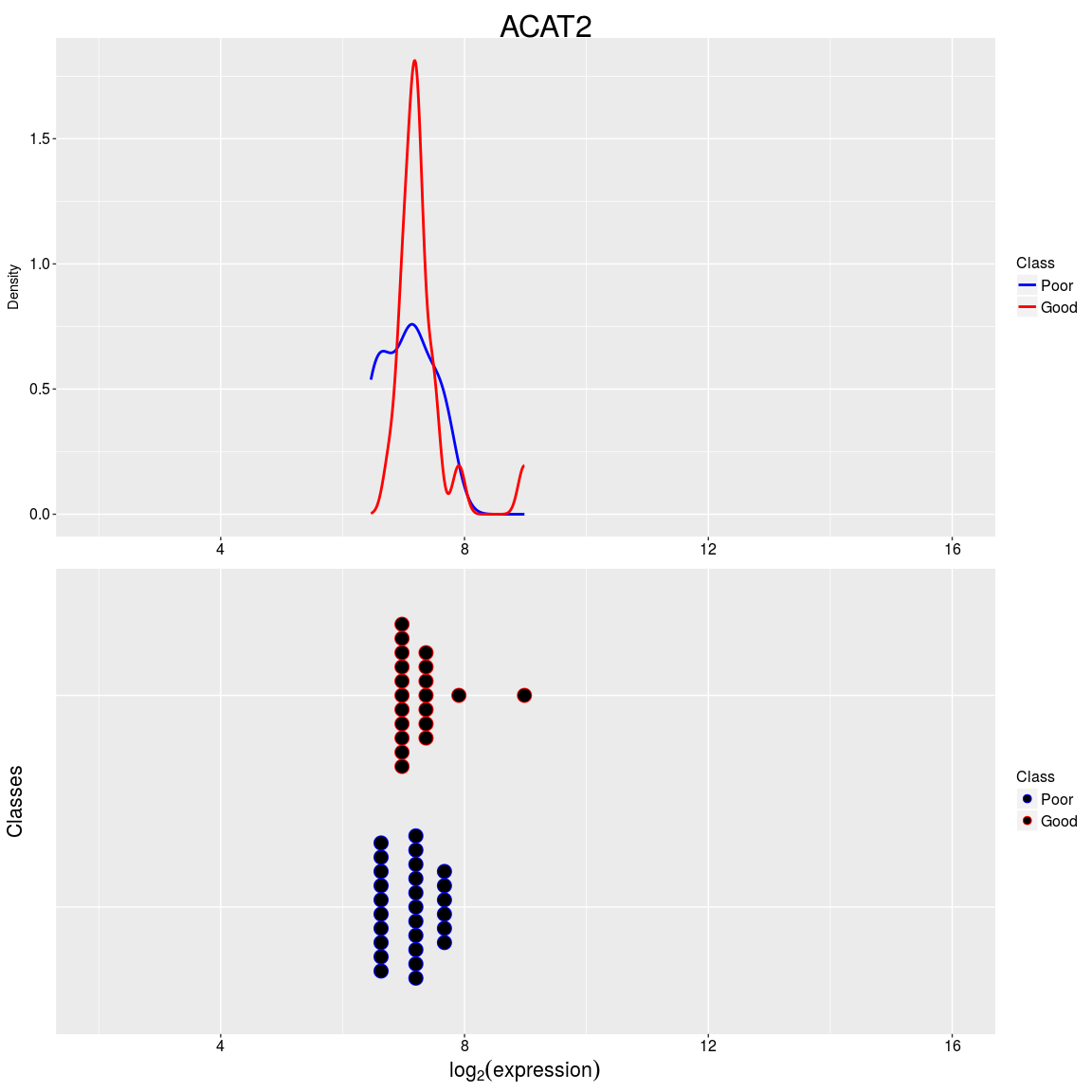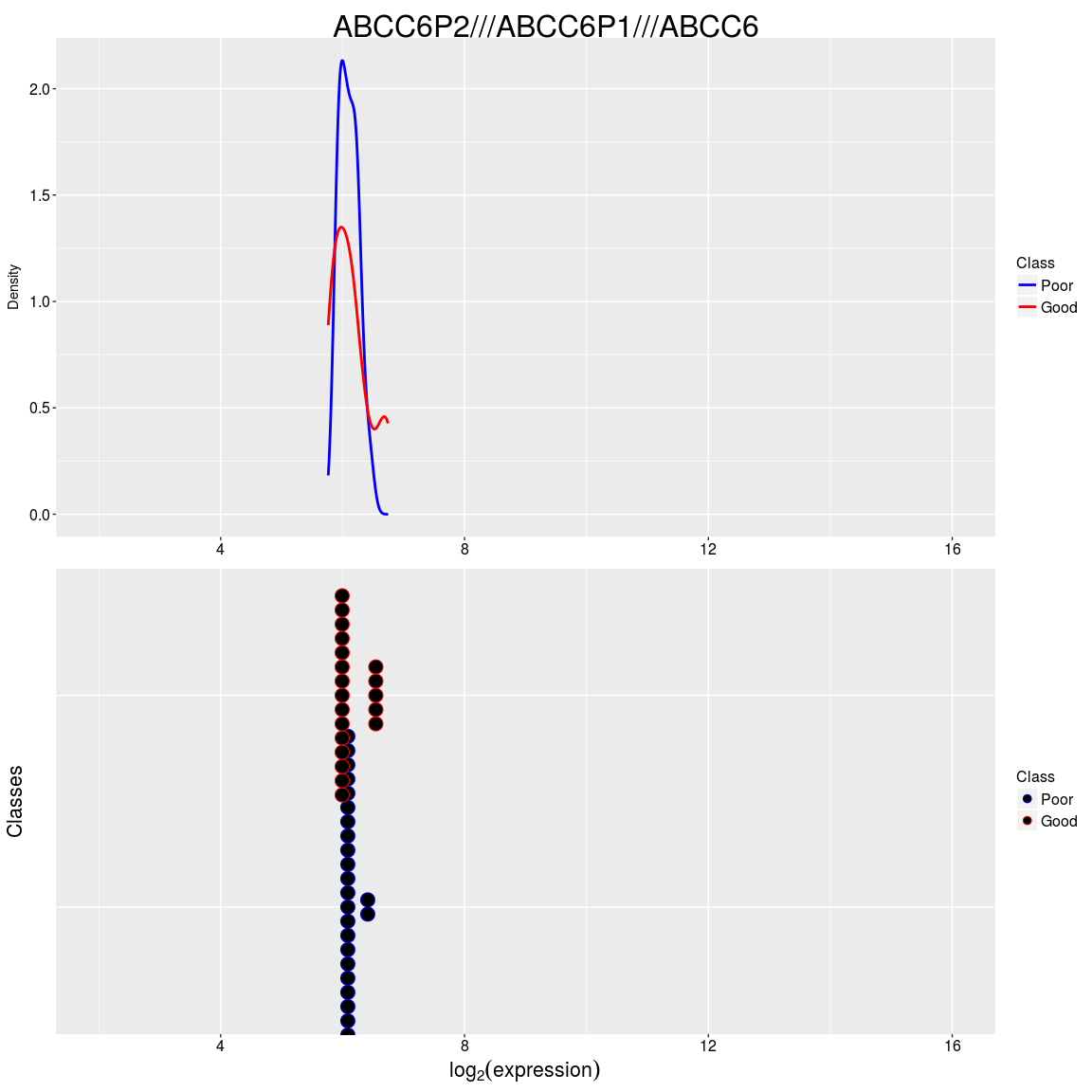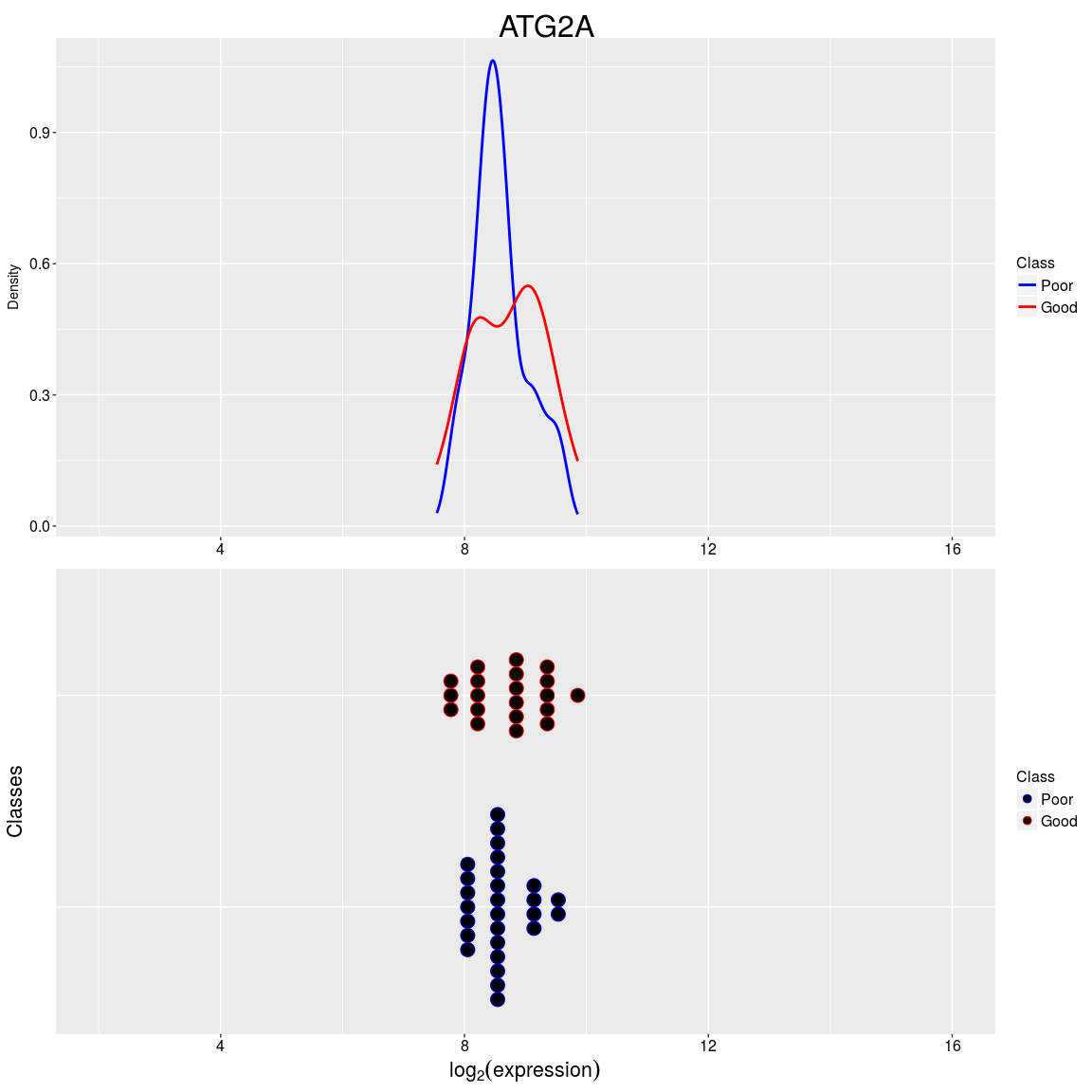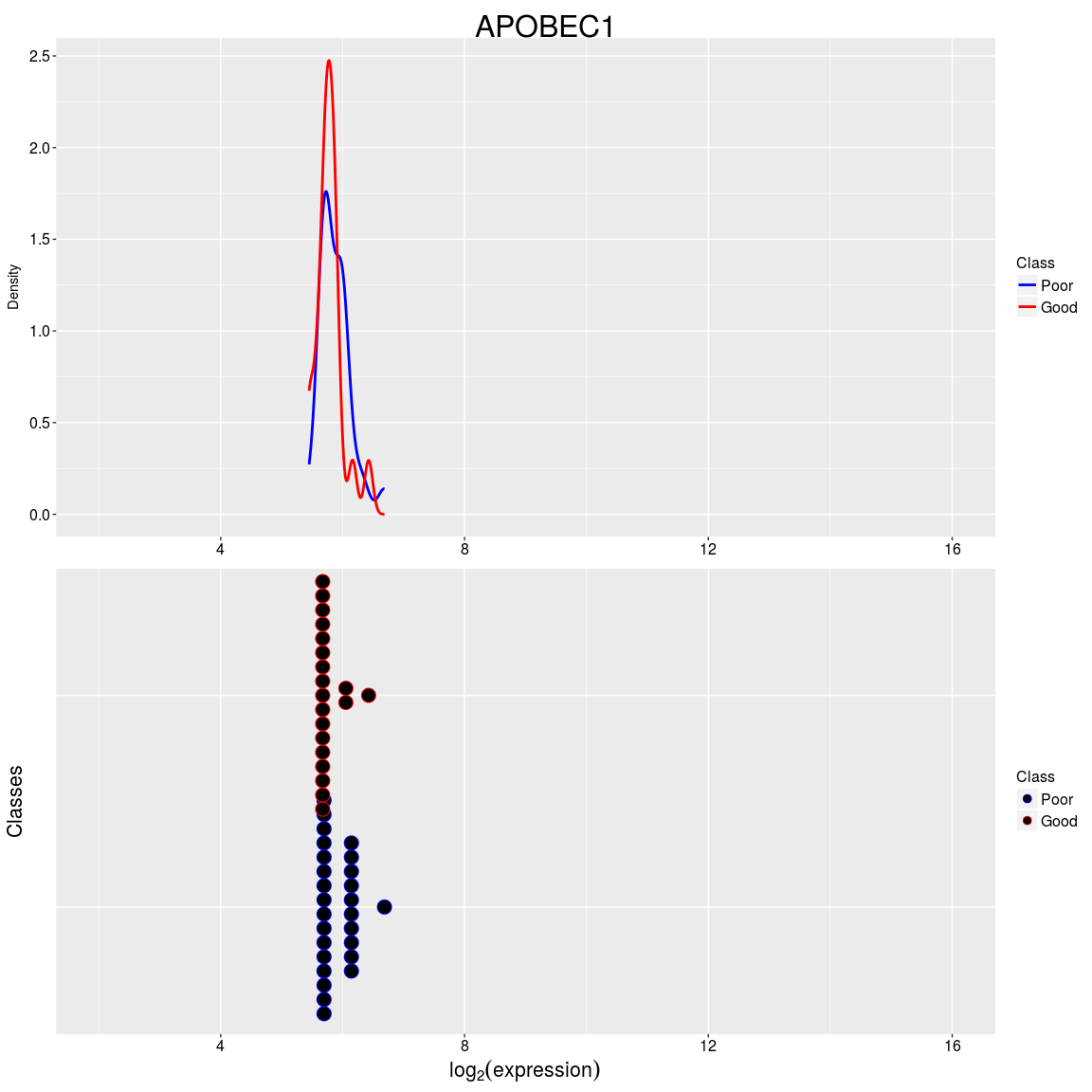Calculate the balanced error rate for differential deviation.

``````DVresults <- calcPerformance(DVresults, "balanced")
DVresults``````
``````## An object of class 'ClassifyResult'.
## Dataset Name: Ovarian Cancer.
## Classification Name: Differential Variability.
## Feature Selection Name: Levene Test.
## Features: List of length 2 of lists of length 4 of row indices.
## Validation: 2 Resamples, 4 Folds.
## Predictions: List of data frames of length 2.
## Performance Measures: Balanced Error Rate.``````
``performance(DVresults)``
``````## \$`Balanced Error Rate`
##  0.1245421 0.2445887``````

The errors are reasonable.

### 0.3.3 Differential Distribution

Differential distribution describes classification based on differences in either the location, scale, or both aspects of a distribution.

ClassifyR has four feature selection functions for differential distribution :

• Likelihood ratio statistic.
• Kolmogorov-Smirnov distance.
• Kullback-Leibler distance.
• Differences of Means/Medians and Deviations (DMD).

There are also two classifiers included : * Naive Bayes. * Mixtures of normals.

Kullback-Leibler divergence will be used for feature selection and a naive Bayes classifier will fit a density to expression values of a gene, for each class. The prediction is then the differences in density between classes, scaled for the number of samples that were in each class in the training set, summed for all selected probes.

``````dParams <- list(bw = "nrd0", n = 4096, from = expression(min(featureValues)),
to = expression(max(featureValues)))
DDresults <- runTests(ovarSet, "Ovarian Cancer", "Differential Distribution",
validation = "bootstrap", resamples = 2, folds = 2,
params = list(SelectParams(KullbackLeiblerSelection, resubstituteParams = ResubstituteParams(nFeatures = c(25, 50, 75, 100), performanceType = "balanced", better = "lower")),
TrainParams(naiveBayesKernel, FALSE, doesTests = TRUE),
PredictParams(predictor = function(){}, FALSE, getClasses = function(result) result, weighted = "weighted", returnType = "both",
densityParameters = dParams)),
verbose = 1)
DDresults``````
``````## \$`weight=crossover distance`
## An object of class 'ClassifyResult'.
## Dataset Name: Ovarian Cancer.
## Classification Name: Differential Distribution.
## Feature Selection Name: Kullback-Leibler Divergence.
## Features: List of length 2 of lists of length 2 of row indices.
## Validation: 2 Resamples, 2 Folds.
## Predictions: List of data frames of length 2.
## Performance Measures: None calculated yet.
##
## \$`weight=height difference`
## An object of class 'ClassifyResult'.
## Dataset Name: Ovarian Cancer.
## Classification Name: Differential Distribution.
## Feature Selection Name: Kullback-Leibler Divergence.
## Features: List of length 2 of lists of length 2 of row indices.
## Validation: 2 Resamples, 2 Folds.
## Predictions: List of data frames of length 2.
## Performance Measures: None calculated yet.
##
## \$`weight=sum differences`
## An object of class 'ClassifyResult'.
## Dataset Name: Ovarian Cancer.
## Classification Name: Differential Distribution.
## Feature Selection Name: Kullback-Leibler Divergence.
## Features: List of length 2 of lists of length 2 of row indices.
## Validation: 2 Resamples, 2 Folds.
## Predictions: List of data frames of length 2.
## Performance Measures: None calculated yet.``````

Since naiveBayesKernel does both training and testing, an empty function is specified as the predictor function for the PredictParams constructor.

The top five probes selected for the first resampling and first fold are visualised.

``DDplots <- plotFeatureClasses(ovarSet, features(DDresults[])[][][1:5])``